# Principal Component Analysis

## What is Principal Component Analysis (PCA)?

Before we go into the technical definition of Principal component analysis (PCA), let us consider a scenario where you want to predict the real-estate pricing of a metropolitan city in the year 2021. You will have lots of information and factors affecting the pricing of the real estate: the land cost, land availability, variations in pricing depending on the locality, interest rates, demographics, government policies and subsidies, economic indicators such as GDP, employment data, prices of goods, traffic conditions,  personal preferences such as availability of basic facilities within a periphery, electricity prices, etc. In short, there are a lot of variables to consider.

Working with a lot of variables can cause the following problems:

• Difficulty in understanding the relationships between variables.
• Likelihood of overfitting your model to your data because of a large number of variables, or you might end up violating assumptions of whichever modeling tactic you're using.
• Do you need to consider all the variables you have collected or target only some of them?

In technical terms, we want to reduce the dimensions of our feature space. By reducing the dimensions of our feature space, we have fewer relationships between variables to consider, which means that we are less likely to overfit our model.

This process of reducing dimensions of our feature space is referred to as dimensionality reduction. It can be achieved in many ways, but most techniques fall under one of these two classes.

• Feature Elimination
• Feature Extraction

## Feature Elimination

In this technique, we reduce the feature space by eliminating the features. In the real-estate example above, we might drop all the variables except the three, which we think will best predict what the prices of real-estate might be. By using feature elimination techniques, we can achieve simplicity and ease in maintaining the interpretability of our variables; on the contrary, by using these techniques, we will gain no information from those variables we've dropped. If we again consider the real-estate pricing prediction, using the availability of essential needs near the land under consideration can significantly vary the demand and hence, the price of the land. If we are using this technique of elimination, we are losing out on the information that these factors can provide us.

## Feature Extraction

Let's assume we have ten independent variables. In feature extraction, we have to create ten "new" independent variables, where every newly created independent variable is a combination of each of the ten of our original independent variables. However, these new independent variables are created in a specific way and are ordered on the basis of "how well they predict our dependent variable?". Now that we have ordered our variables by how well they predict our dependent variable, we know which variable is most and least important, so we can rapidly drop off the least significant ones. But because of the fact that the new independent variables are a combination of our old ones, we are still retaining the fundamental attributes of our old variables.

The principal component analysis is a technique for feature extraction, in which it combines our input variables in a specific way so that we can drop the "least important/ least significant" variables while still retaining the fundamental attributes of our old variables.

## How does Principal component Analysis (PCA) work?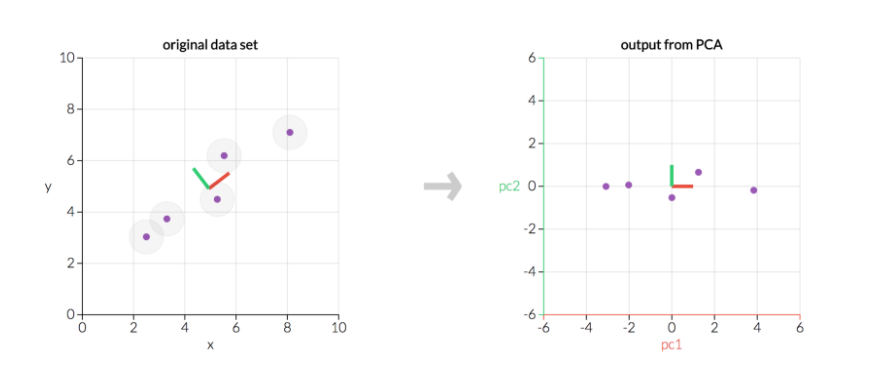Here, we transform five data points using Principal component analysis (PCA). The left graph is our original data; the right graph would be our transformed data.

source: setosa.io

Principal Component analysis can be broken down into five steps:

1. Standardization of the range of continuous initial variables
2. Computation of the covariance matrix to identify correlations
3. Computation of the eigenvectors and eigenvalues of the covariance matrix to identify the principal components
4. Creation of a feature vector to decide which principal components to keep
5. Recasting the data along the axes of the principal component

### Step 1: Standardization

In this step, we aim to bring all the variables to one standard range so that each one of them contributes equally to the analysis. The difference in the ranges of the variables will result in the dominance of variables with more significant differences within their ranges over the ones which have smaller ranges. For instance, a variable that ranges between 0 and 1000 will be dominant over a variable that has a range between 0 and 1, and this will lead to biased results. So, we will have to transform the data to comparable scales to prevent this problem.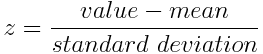### Step 2: Covariance Matrix Computation

The main aim of this step is to understand how the variables of the input data set vary from the mean with respect to each other, that is, to determine if there exists any relationship between them. The covariance matrix is a [n × n] symmetric matrix (where n is the number of dimensions) that has as entries the covariances related to all possible pairs of the initial variables.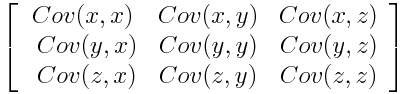What do the covariance matrix entries tell us about the correlations between the variables?

It is actually the sign of the covariance that matters :

• if  the sign is positive: the two variables increase or decrease together (correlated)
• if the sign is negative: One variable increases when the other decreases (Inversely correlated)

### Step 3: Computing the eigenvectors and eigenvalues of the covariance matrix.

Eigenvectors and eigenvalues are the linear algebra concepts, which are required to be computed from the covariance matrix to determine the principal components of the data, which are the "new" variables that are formed from linear combinations or mixtures of the initial variables in our feature set.

Let's assume that the eigenvectors and values of the covariance matrix of our data set are 2-dimensional with two variables (x,y)  are as follows: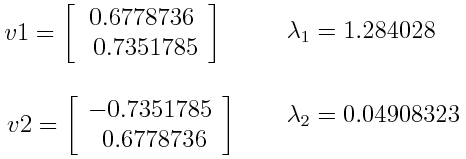### Step 4: Creation of a feature vector

In this step, we decide if we want to keep all these attributes or discard those of lesser significance (i.e., of low eigenvalues) and, with the help of the remaining ones, form a matrix of vectors that we call Feature vectors.

This will reduce the dimensions of our feature set because if we choose to keep only p eigenvectors (components) out of n, the final data set will have only p dimensions.

### Step 5: Recast the data along the axes of the principal component

In this step, we use the feature vector formed using the eigenvectors of the covariance matrix to reorient the data from the original axes to those represented by the principal components (hence the name Principal Components Analysis). To do this, we multiply the transpose of the original data set by the transpose of the feature vector.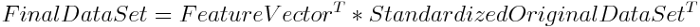1). When should we use Principal component analysis (PCA)?

• When we want to reduce the number of variables, we aren't able to identify the variables which can be removed entirely from consideration.
• When we want to ensure your variables are independent of one another
• If we're going to make our independent variables less interpretable

2). What are the limitations of Principal component analysis (PCA)?

• Even though principal components are the linear combination of the attributes of the original variables, they are not very easy to interpret.
• It's a trade-off between information loss and dimensionality reduction.

3). What type of data should be used for Principal component analysis (PCA)?

Principal component analysis (PCA) works best on a data set having three or more dimensions. Because, with more dimensions, it becomes increasingly challenging to make interpretations from the resultant cloud of data.

## Key Takeaways

If we have a lot of independent variables to handle, we use Principal component analysis (PCA) to reduce the dimensions of our feature set.

The principal component analysis is a technique, which combines our input variables through their linear combinations and mixtures, and then we can drop the "least significant" variables while still retaining the most valuable attributes of all of the variables.